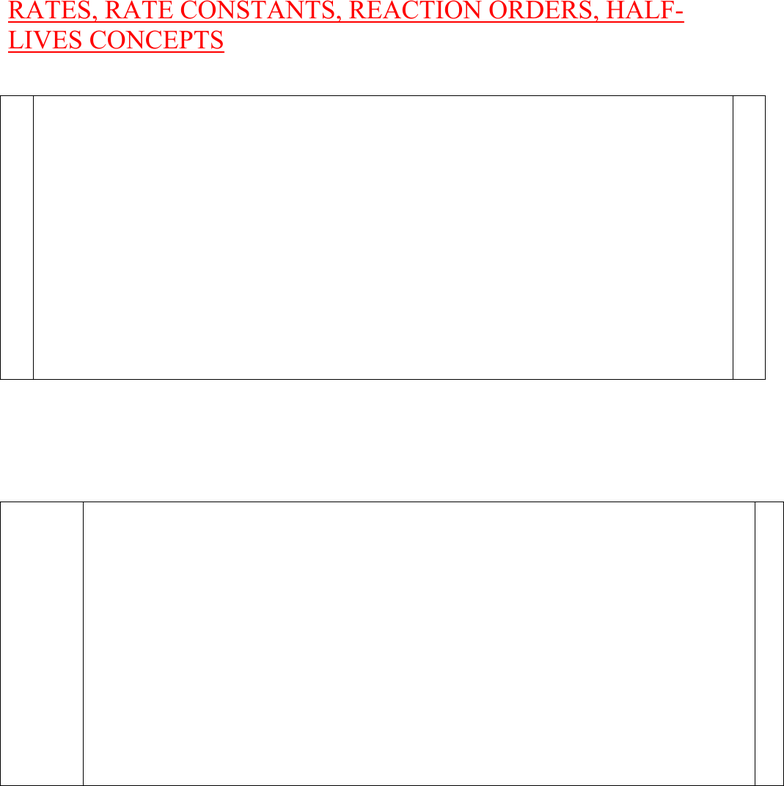Study Guides (380,000)
US (220,000)
Rutgers (3,000)
3:40 (100)
Final

01:160:161 Chapter Notes - Chapter 15: Half-Life, Reaction Rate Constant, Reaction MechanismExam

Department
Chemistry
Course Code
01:160:161
Professor
Asbed Vassilia
Study Guide
Final

This preview shows pages 1-3. to view the full 46 pages of the document.1
PRACTICE PROBLEMS
CHEM 162-2016
CHAPTER 15 – CHEMICAL KINETICS
RATES, RATE CONSTANTS, REACTION ORDERS, HALF-LIVES CONCEPTS
RATES,RATE CONSTANTS, REACTION ORDERS, HALF-LIVES CALCULATIONS
REACTION MECHANISMS, ACTIVATION ENERGY & CATALYSTS CONCEPTS
REACTION MECH., ACTIVATION ENERGY & CATALYSTS CALCULATIONS
E. Tavss, PhD

Only pages 1-3 are available for preview. Some parts have been intentionally blurred.2
RATES, RATE CONSTANTS, REACTION ORDERS, HALF-
LIVES CONCEPTS
12 Chem 162-2011 Final exam
Chapter 13a - Kinetics
Rates, rate constants, reaction orders, half-lives concepts
The rate law for a reaction is rate = k [A]2[B]
Which of the following statements is FALSE?
A. The reaction is second order in A
B. The reaction is first order in B
C. k is the reaction rate constant
D. If the concentration of A is doubled, the reaction rate will quadruple
E. The reaction is second order overall
17 Chem 162-2011 Exam I
Hill & Petrucci et al. 4th edition
Chapter 13A – Kinetics
Rates, rate constants, reaction orders, half-lives concepts
A graph of 1/[A] vs. time gives a straight line. Which rate law is consistent with this graph?
A. r = k[A]2
B. r = k
C. r = k[A]
D. r = k[A]-1
E. r = k[A]3

Only pages 1-3 are available for preview. Some parts have been intentionally blurred.3
22 Chem 162-2011 Exam I
Hill & Petrucci et al. 4th edition
Chapter 13A – Kinetics
Rates, rate constants, reaction orders, half-lives concepts
Which units of k are consistent with a fifth order rate law?
A. M-5 s-1
B. M-4 s-1
C. M4 s-1
D. M-4 s-4
E. M s-1
23 Chem 162-2011 Exam I
Hill & Petrucci et al. 4th edition
Chapter 13A – Kinetics
Rates, rate constants, reaction orders, half-lives concepts
Consider the reaction 4NH3(g) + 5O2(g) 4NO(g) + 6H2O(g). If the rate of disappearance
of O2 is 0.145 atm/s, what is the rate of appearance of H2O?
A. 0.145 atm/s
B. 0.121 atm/s
C. 0.0290 atm/s
D. 0.870 atm/s
E. 0.174 atm/s
CHEM 162-2010 EXAM I
Chapter 13A - Kinetics
Rates, rate constants, reaction orders, half-lives concepts
20. Given the reaction A + 3B 2C + D, if the rate of appearance of C is 0.015 M/s, what is the rate of
disappearance of B?
A. 0.010 M/s
B. 0.0050 M/s